## Interference of Spring Waves

Velocity of Periodic Waves

We have learned that, if the medium is constant than velocity of the wave is also constant and we gave following equations for velocity of waves;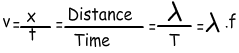Example:  Find the relation of wavelengths of given waves.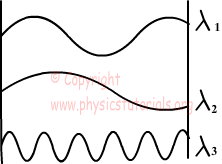We define wavelength as the distance between the sequential crests or troughs.Picture given below shows the wavelengths of each wave and relation between them.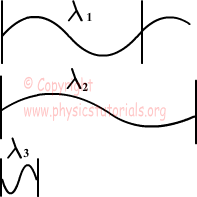Example: Using the data given in the picture below; find the wavelength, velocity and amplitude of the wave. Frequency of the source is 2s-1.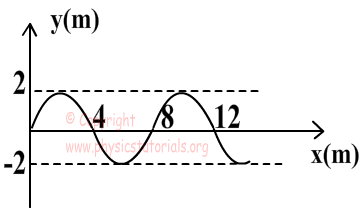We find wavelength of the wave from the picture as;

8m

We find velocity of the wave by using the following formula;

v=wavelength.freguency=8m.2s-1=16m/s

Amplitude of the wave is 2m from the given picture.

Interference of Spring Waves

When two waves interfere they produce resultant wave. In this section we learn how to find resultant wave. Displacement of the resultant wave is the sum of the waves producing it. Look at the given pictures below. They show the behavior of the waves before the interference, and after the interference.

Example: Amplitudes of the waves given below are A1 and A2. Picture shows  the interference of two waves.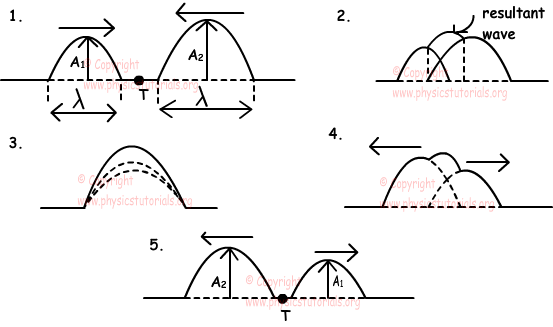Picture given below shows two identical waves interference having opposite directions.Be Careful!

If the amplitudes of the waves having opposite directions are different, then amplitude of the resultant wave becomes the difference of the amplitudes of waves. It has the same direction with the bigger wave.

Waves Exams and Solutions

Author:

Tags: